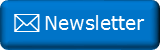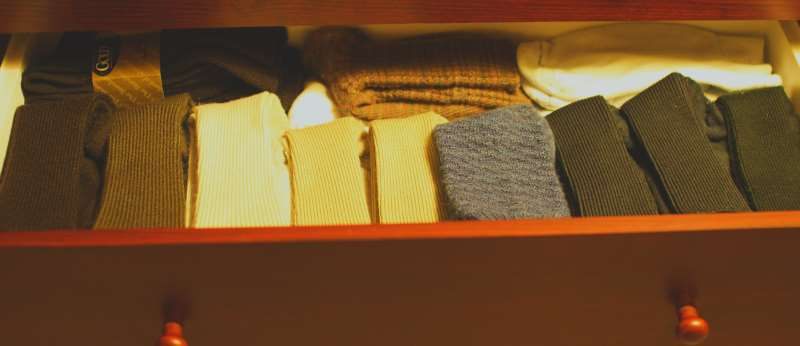﻿ Sock Puzzle RevisitedNewsletter: Click here to join an email list and be contacted when a new article is published.      Advertisement

# Sock Puzzle Revisited

There’s a classic critical thinking problem popular in schools and puzzle books. It’s stated in various forms, but here’s the gist:

In your sock drawer, you have a dozen socks: A half-dozen white socks, and a half-dozen red socks. You are about to pull out a pair of socks when the power goes out, plunging the entire room into darkness. What is the minimum number of socks you should take from the drawer to ensure you get a matching set of socks to wear?Image: Mr.TinDC

Implicit in this puzzle are premises that:

• All of the socks are stored singly.

• All the socks are randomly distributed in the drawer.

• There is no difference between a right sock and a left sock.

The answer is, of course, three socks. (The first two socks could match, but if not, the third sock is guaranteed to match one of the others).Above are all eight possible combinations of socks possible.

### Specific MatchAn extension question: “How many socks would you need to take to guarantee you get a pair of white socks to wear?” This is a little more challenging, but not much. The answer is eight. (In the worst case, your first six pulls could all be red socks. After this, there’s only white left so just two more is all that is necessary to guarantee a white pair).

## Pulling in pairsNow let’s change the problem slightly. Imagine you pull out the socks in pairs, check them with a flash-light, set them aside, then return to the drawer and pull out another pair, then again, and again, until all the socks have been taken.

Questions:

• What’s the probability that every pair you pull out will be a mismatch? (One red sock and one white).

• What’s the probability that every pair you pull out will match?

Do you have any intuition as to which will be more likely?

Let's do the math for the generic solution …

## Every pair a mismatch

If there a n socks of each colour in the drawer, this means there are 2n socks in total.

(e.g.With six red socks, and six white socks, there are a total of 12 individual socks).

The way we can think of this puzzle as "Draw a sock", then another, then another (without replacement) until there are none left.

The number of ways these socks can be pulled this way is: (2n)!

What we need to calculate, for all these combinations, is the number that are mismatched. The ratio of these two numbers is the probability.

For the first pull, there are (2n) possible socks (any of the socks), for the second pull, however it has to be from the other set, so there are (n) combinations. On the next pull, there are (2n-2) combinations, followed by (n-1)

This continues in pairs, all the way down to 2 and 1 socks. Odd pulls can be any of the remaining socks, even pulls combinations are from half the remaining. Multiplying all these gives the number of possible solutions (Numerator) that match the criteria we need. The total of all possible combinations is the the Denominator

Numerator = (2n)(n)(2n-2)(n-1)(2n-4)(n-2)(2n-6)(n-3) … (2)(1)

Grouping the odd and even terms:

Numerator = (2n)(2n-2)(2n-4)(2n-6)…(2) × (n)(n-1)(n-2)(n-3)…(1)

Numerator = 2(n)2(n-1)2(n-2)2(n-3)…2(1) × (n)(n-1)(n-2)(n-3)…(1)

Numerator = (2n)[(n)(n-1)(n-2)(n-3)…(1)] × (n)(n-1)(n-2)(n-3)…(1)

Simplifying:

Numerator = 2n×(n!)×(n!) = 2n(n!)2

We already know the Denominator is (2n)!

The probability is therefore:

Prall_mismatched = 2n(n!)2/(2n)!

## Every pair a match

This is a little more complicated. First, it's only possible if there are an even number of each colour. If we had n=3 (meaning we had three red socks, and three white socks), there's no way we can pull all the socks in pairs! After we pulled out the first pair on any colour, there would be an, unloved, singleton remaining.

We also need a different strategy to solve this situation, as socks are not pulled out decreasing in count in pairs. As long as the pairs match they can be pulled out in any order (for instance, it's possible to pull out all the red pairs first, then all the white, or in any combination in-between).

Let's imagine pulling the socks out in pairs. As we have n socks of each colour (with n being even) then there are n pairs of socks that will be pulled out of the drawer.

For the n red socks, there are nCn/2 (n choose n/2) ways to these pairs can the arranged (the other pairs, by definition being white pairs).

As an example, let's imagine we have n=6, so there are six red socks and six white socks. If we pulled them out in pairs, there will be six pairs.For n=6, there are a total of 6C3 = 20 different ways these pairs can be arranged:For each of these nCn/2 ways to pull pairs, there are n! ways the n red socks can be arranged, and n! ways the n white socks can be arranged.

Numerator = nCn/2 × n! × n!    [where n is even]

The Denominator is the same as before: (2n)!

The probability is therefore:

Prall_matched = nCn/2(n!)2/(2n)!

## Data

Here are results for the first few values of n:

nPrmismatchPrmatched
1100.000%
266.667%33.333%
340.000%
422.857%8.571%
512.698%
66.926%2.165%
73.730%
81.989%0.544%
91.053%
100.554%0.136%

A quick sanity check shows this is what is expected. If there is just one of each sock n=1, then it's 100% certain they will be mismatched (and undefined to pull them out as matching pair!) If there are two red and two white n=2, then whatever the first sock, there are three left to choose from; two of these (2/3) will cause a mismatch for the first pair (guaranteeing the second pair will mismatch too). One of them (1/3) will match (and also guarantee the second pair will match).

Going back to the original question of six red and six white. There is 6.926% chance all drawn pairs will mismatch, and a 2.165% chance that they will all match.

It's always more likely that all the pairs will be mismatched cf. all pairs matching.

You can find a complete list of all the articles here.Click here to receive email alerts on new articles.